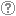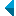# Search the repositoryQuery: search in TitleAuthorAbstractKeywordsFull textYear of publishing ANDORAND NOT search in TitleAuthorAbstractKeywordsFull textYear of publishing ANDORAND NOT search in TitleAuthorAbstractKeywordsFull textYear of publishing ANDORAND NOT search in TitleAuthorAbstractKeywordsFull textYear of publishing Work type: All work types Habilitation (m4) Specialist thesis (m3) High school thesis (m6) Bachelor work * (dip) Master disertations * (mag) Doctorate disertations * (dok) Research Data or Corpuses (data) * old and bologna study programme Language: All languagesSlovenianEnglishGermanCroatianSerbianBosnianBulgarianCzechFinnishFrenchGerman (Austria)HungarianItalianJapaneseLithuanianNorwegianPolishRussianSerbian (cyrillic)SlovakSpanishSwedishTurkishUnknown Search in: RUP    FAMNIT - Faculty of Mathematics, Science and Information Technologies    FHŠ - Faculty of Humanities    FM - Faculty of Management    FTŠ Turistica - Turistica – College of Tourism Portorož    FVZ - Faculty of Health Sciences    IAM - Andrej Marušič Institute    PEF - Faculty of Education    UPR - University of Primorska    ZUP - University of Primorska PressCOBISS    Fakulteta za humanistične študije, Koper    Fakulteta za management Koper in Pedagoška fakulteta Koper    Fakulteta za vede o zdravju, Izola    Knjižnica za tehniko, medicino in naravoslovje, Koper    Turistica, Portorož    Znanstveno-raziskovalno središče Koper Options: Show only hits with full text Reset

 1 - 8 / 811.Rank-permutable additive mappingsAleksandr Èmilevič Guterman, Anna A. Alieva, Bojan Kuzma, 2006, original scientific articleAbstract: Let ▫$\sigma$▫ be a fixed non-identical permutation on ▫$k$▫ elements. Additive bijections ▫$T$▫ on the matrix algebra ▫$M_n(\mathbb{F})$▫ over a field ▫$\mathbb{F}$▫ of characteristic zero, with the property that ▫$\rm{rk} (A_1...A_k) = \rm{rk} (A_{\sigma(1)}...A_{\sigma(k)})$▫ implies the same condition on the ▫$T$▫ images, are characterized. It is also shown that the surjectivity assumption can be relaxed, if this property is preserved in both directions.Found in: ključnih besedahSummary of found: ...▫$k$▫ elements. Additive bijections ▫$T$▫ on the matrix algebra ▫$M_n(\mathbb{F})$▫ over a field ▫$\mathbb{F}$▫ of...Keywords: mathematics, linearna algebra, matrix algebra, rank, permutation, additive preserversPublished: 15.10.2013; Views: 2632; Downloads: 85Full text (0,00 KB) 2.Q-polynomial distance-regular graphs with a [sub] 1 [equal] 0 and a [sub] 2 [not equal] 0Štefko Miklavič, 2008, original scientific articleAbstract: Let ▫$\Gamma$▫ denote a ▫$Q$▫-polynomial distance-regular graph with diameter ▫$D \ge 3$▫ and intersection numbers ▫$a_1=0$▫, ▫$a_2 \ne 0$▫. Let ▫$X$▫ denote the vertex set of ▫$\Gamma$▫ and let ▫$A \in {\mathrm{Mat}}_X ({\mathbb{C}})$▫ denote the adjacency matrix of ▫$\Gamma$▫. Fix ▫$x \in X$▫ and let denote $A^\ast \in {\mathrm{Mat}}_X ({\mathbb{C}})$ the corresponding dual adjacency matrix. Let ▫$T$▫ denote the subalgebra of ▫$A{\mathrm{Mat}}_X ({\mathbb{C}})$▫ generated by ▫$A$▫, ▫$A^\ast$▫. We call ▫$T$▫ the Terwilliger algebra of ▫$\Gamma$▫ with respect to ▫$x$▫. We show that up to isomorphism there exists a unique irreducible ▫$T$▫-module ▫$W$▫ with endpoint 1. We show that ▫$W$▫ has dimension ▫$2D-2$▫. We display a basis for ▫$W$▫ which consists of eigenvectors for ▫$A^\ast$▫. We display the action of ▫$A$▫ on this basis. We show that ▫$W$▫ appears in the standard module of ▫$\Gamma$▫ with multiplicity ▫$k-1$▫, where ▫$k$▫ is the valency of ▫$\Gamma$▫.Found in: ključnih besedahSummary of found: ...▫$A \in {\mathrm{Mat}}_X ({\mathbb{C}})$▫ denote the adjacency matrix of ▫$\Gamma$▫. Fix ▫$x \in X$▫ and... ...dual adjacency matrix. Let ▫$T$▫ denote the sub algebra of ▫$A{\mathrm{Mat}}_X ({\mathbb{C}})$▫ generated by ▫$A$▫, ▫$A^\ast$▫....Keywords: mathematics, graph theory, adjacency matrix, distance-regular graph, Terwilliger algebraPublished: 15.10.2013; Views: 3251; Downloads: 27Full text (0,00 KB) 3.Jordan [tau]-derivations of locally matrix ringsAjda Fošner, Chen-Lian Chuang, Tsiu Kwen Lee, 2013, original scientific articleAbstract: Let ▫$R$▫ be a prime, locally matrix ring of characteristic not 2 and let ▫$Q_{ms}(R)$▫ be the maximal symmetric ring of quotients of ▫$R$▫. Suppose that ▫$\delta \colon R \to Q_{ms}(R)$▫ is a Jordan ▫$\tau$▫-derivation, where ▫$\tau$▫ is an anti-automorphism of $R$. Then there exists ▫$a \in Q_{ms}(R)$▫ such that ▫$\delta(x) = xa - a\tau(x)$▫ for all ▫$x \in R$▫. Let ▫$X$▫ be a Banach space over the field ▫$\mathbb{F}$▫ of real or complex numbers and let ▫$\mathcal{B}(X)$▫ be the algebra of all bounded linear operators on ▫$X$▫. We prove that ▫$Q_{ms}(\mathcal{B}(X)) = \mathcal{B}(X)$▫, which provides the viewpoint of ring theory for some results concerning derivations on the algebra ▫$\mathcal{B}(X)$▫. In particular, all Jordan ▫$\tau$▫-derivations of ▫$\mathcal{B}(X)$▫ are inner if ▫$\dim_{\mathbb{F}} X>1$▫.Found in: ključnih besedahSummary of found: ...complex numbers and let ▫$\mathcal{B}(X)$▫ be the algebra of all bounded linear operators on ▫$X$▫....Keywords: mathematics, algebra, anti-automorphism, locally matrix ring, prime ring, Jordan homomorphism, Jordan ▫$\tau$▫-derivation, Banach spacePublished: 15.10.2013; Views: 2840; Downloads: 76Full text (0,00 KB) 4.Jordan triple product homomorphismsBojan Kuzma, 2006, original scientific articleAbstract: Nondegenerate mappings that preserve Jordan triple product on ▫${\mathscr{M}}_n({\mathbb{F}}$▫ are characterized. Here, ▫$n \ge 3$▫ and ▫$\mathbb{F}$▫ is an arbitrary field.Found in: ključnih besedahSummary of found: ...mathematics, linear algebra, matrix algebra, Jordan triple product, nonlinear preserver...Keywords: mathematics, linear algebra, matrix algebra, Jordan triple product, nonlinear preserverPublished: 15.10.2013; Views: 3237; Downloads: 134Full text (0,00 KB) 5.Mappings that preserve pairs of operators with zero triple Jordan productGorazd Lešnjak, Bojan Kuzma, Mirko Dobovišek, Chi-Kwong Li, Tatjana Petek, 2007, original scientific articleAbstract: Let ▫$\mathbb{F}$▫ be a field and ▫$n \ge 3$▫. Suppose ▫${\mathfrak{G_1,G_2}} \subseteq M_n(\mathbb{F})▫$ contain all rank-one idempotents. The structure of surjections ▫$\phi : \mathfrak{G_1} \to \mathfrak{G_2}$▫ satisfying ▫$ABA = 0 \iff \phi(A)\phi(B)\phi(A) = 0$▫ is determined. Similar results are also obtained for (a) subsets of bounded operators acting on a complex or real Banach space, (b) the space of Hermitian matrices acting on ▫$n$▫-dimensional vectors over a skew-field, (c) subsets of self-adjoint bounded linear operators acting on an infinite dimensional complex Hilbert space. It is then illustrated that the results can be applied to characterize mappings ▫$\phi$▫ on matrices or operators such that ▫$F(ABA) = F(\phi(A)\phi(B)\phi(A))▫$ for all ▫$A,B$▫ for functions ▫$F$▫ such as the spectral norm, Schatten ▫$p$▫-norm, numerical radius and numerical range, etc.Found in: ključnih besedahSummary of found: ... matrix algebra, Jordan triple product, nonlinear preservers...Keywords: matrix algebra, Jordan triple product, nonlinear preserversPublished: 03.04.2017; Views: 1830; Downloads: 93Full text (0,00 KB) 6.General preservers of quasi-commutativityGregor Dolinar, Bojan Kuzma, 2010, original scientific articleAbstract: Let ▫$M_n$▫ be the algebra of all ▫$n \times n$▫ matrices over ▫$\mathbb{C}$▫. We say that ▫$A, B \in M_n$▫ quasi-commute if there exists a nonzero ▫$\xi \in \mathbb{C}$▫ such that ▫$AB = \xi BA$▫. In the paper we classify bijective not necessarily linear maps ▫$\Phi \colon M_n \to M_n$▫ which preserve quasi-commutativity in both directions.Found in: ključnih besedahSummary of found: ...mathematics, linear algebra, general preserver, matrix algebra, quasi-commutativity...Keywords: mathematics, linear algebra, general preserver, matrix algebra, quasi-commutativityPublished: 03.04.2017; Views: 1412; Downloads: 75Full text (0,00 KB) 7.On maximal distances in a commuting graphBojan Kuzma, Gregor Dolinar, Polona Oblak, 2012, original scientific articleAbstract: It is shown that matrices over algebraically closed fields that are farthest apart in the commuting graph must be non-derogatory. Rank-one matrices and diagonalizable matrices are also characterized in terms of the commuting graph.Found in: ključnih besedahSummary of found: ...mathematics, linear algebra, graph theory, commuting graph, matrix algebra, algebraically closed field, centralizer, distance in...Keywords: matematika, linearna algebra, teorija grafov, komutirajoči grafi, matrična algebra, algebraično zaprt obseg, centralizator, razdalja v grafih, mathematics, linear algebra, graph theory, commuting graph, matrix algebra, algebraically closed field, centralizer, distance in graphsPublished: 03.04.2017; Views: 1712; Downloads: 230Full text (0,00 KB)This document has more files! More... 8.Permanent versus determinant over a finite fieldAleksandr Èmilevič Guterman, Gregor Dolinar, Bojan Kuzma, Marko Orel, 2013, published scientific conference contributionAbstract: Let ▫$\mathbb{F}$▫ be a finite field of characteristic different from 2. We study the cardinality of sets of matrices with a given determinant or a given permanent for the set of Hermitian matrices ▫$\mathcal{H}_n(\mathbb{F})$▫ and for the whole matrix space ▫$M_n(\mathbb{F})$▫. It is known that for ▫$n = 2$▫, there are bijective linear maps ▫$\Phi$▫ on ▫$\mathcal{H}_n(\mathbb{F})$▫ and ▫$M_n(\mathbb{F})$▫ satisfying the condition per ▫$A = \det \Phi(A)$▫. As an application of the obtained results, we show that if ▫$n \ge 3$▫, then the situation is completely different and already for ▫$n = 3$▫, there is no pair ofmaps ▫$(\Phi, \phi)$▫, where ▫$\Phi$▫ is an arbitrary bijective map on matrices and ▫$\phi \colon \mathbb{F} \to \mathbb{F}$▫ is an arbitrary map such that per ▫$A = \phi(\det \Phi(A))$▫ for all matrices ▫$A$▫ from the spaces ▫$\mathcal{H}_n(\mathbb{F})$▫ and ▫$M_n(\mathbb{F})$▫, respectively. Moreover, for the space ▫$M_n(\mathbb{F})$▫, we show that such a pair of transformations does not exist also for an arbitrary ▫$n > 3$▫ if the field ▫$\mathbb{F}$▫ contains sufficiently many elements (depending on ▫$n$▫). Our results are illustrated by a number of examples.Found in: ključnih besedahSummary of found: ...Hermitian matrices ▫$\mathcal{H}_n(\mathbb{F})$▫ and for the whole matrix space ▫$M_n(\mathbb{F})$▫. It is known that for... ...mathematics, linear algebra, matrix theory, permanent, determinant...Keywords: mathematics, linear algebra, matrix theory, permanent, determinantPublished: 03.04.2017; Views: 1536; Downloads: 85Full text (0,00 KB)
Search done in 0 sec.